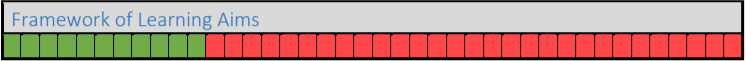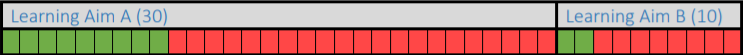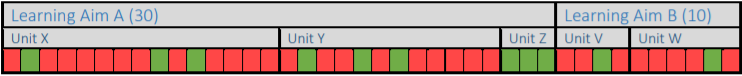Software Update: Wednesday 18th May 2022 - 07:00-07:30am

On Wednesday morning, we're updating OneFile to release some new changes and improvements.
To find out more, our release notes are available
here.
Please ensure you have saved any work and have logged out prior to 7:00am to avoid any potential loss of data.

# How is Progress Calculated?

Progress is tracked and calculated on OneFile by using assessments and selecting criteria.

When an assessment is completed and signed by the assessor and learner, it will count towards progress. The amount of progress made on the assessment is determined by the number of selected criteria and the total number of criteria the learner needs to cover on their portfolio.

Overall Progress

The overall progress shows how much progress has been made towards completing a framework or portfolio of learning aims.

Each coloured box below represents the total number of criteria required to complete the framework. The green boxes indicate the number of criteria covered on an assessment. The red boxes indicate the number of criteria that have not yet been covered.Calculate the percentage made by this assessment

(Number of criteria covered on assessment ÷ Total criteria in Framework) x 100 = progress % (11 ÷ 40) x 100 = 27.5%

Learning Aim Progress

OneFile can also calculate progress for each of the learning aims assigned to a learner.Calculate the percentage made by this assessment for each learning aim

(Number of criteria covered in Learning Aim ÷ Total criteria in Learning Aim) x 100 = progress %

Learning Aim A: (9 ÷ 30) x 100 = 30%         Learning Aim B: (2 ÷ 10) X 100 = 20%

Unit Progress

Progress can also be calculated for each of the units a learner is working towards. When a unit reaches 100%, it can be signed off by the assessor, learner and IV.Calculate the percentage made by this assessment for each unit

(Number of criteria covered in Unit ÷ Total criteria in unit) x 100 = progress %

Learning Aim A

Unit X: (3 ÷ 15) x 100 = 20%        Unit Y: (3 ÷ 12) x 100 = 25%        Unit Z:  (3 ÷ 3) x 100 = 100%

Learning Aim B

Unit V: (1 ÷ 4) x 100 = 25%          Unit W: (1 ÷ 6) x 100 = 17%

Did you find it helpful? Yes No

Send feedback